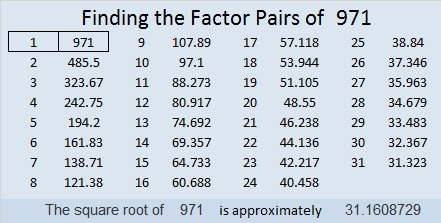# 971 and Level 2

Some of the clues in today’s puzzles are perfect squares. Some aren’t. Can you figure out which are which and put their factors in the right places in the first column and the top row?Print the puzzles or type the solution in this excel file: 10-factors-968-977

971 is palindrome 2D2 in BASE 19 (D is 13 base 10)
because 2(19²) + 13(19) + 2(1) = 971

• 971 is a prime number.
• Prime factorization: 971 is prime.
• The exponent of prime number 971 is 1. Adding 1 to that exponent we get (1 + 1) = 2. Therefore 971 has exactly 2 factors.
• Factors of 971: 1, 971
• Factor pairs: 971 = 1 × 971
• 971 has no square factors that allow its square root to be simplified. √971 ≈ 31.16087

How do we know that 971 is a prime number? If 971 were not a prime number, then it would be divisible by at least one prime number less than or equal to √971 ≈ 31.2. Since 971 cannot be divided evenly by 2, 3, 5, 7, 11, 13, 17, 19, 23, 29 or 31, we know that 971 is a prime number.This site uses Akismet to reduce spam. Learn how your comment data is processed.